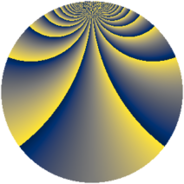# Properties

 Label 648.2.bbLevel $648$ Weight $2$ Character orbit 648.bb Rep. character $\chi_{648}(11,\cdot)$ Character field $\Q(\zeta_{54})$ Dimension $1908$ Newform subspaces $2$ Sturm bound $216$ Trace bound $1$

# Learn more

## Defining parameters

 Level: $$N$$ $$=$$ $$648 = 2^{3} \cdot 3^{4}$$ Weight: $$k$$ $$=$$ $$2$$ Character orbit: $$[\chi]$$ $$=$$ 648.bb (of order $$54$$ and degree $$18$$) Character conductor: $$\operatorname{cond}(\chi)$$ $$=$$ $$648$$ Character field: $$\Q(\zeta_{54})$$ Newform subspaces: $$2$$ Sturm bound: $$216$$ Trace bound: $$1$$ Distinguishing $$T_p$$: $$5$$

## Dimensions

The following table gives the dimensions of various subspaces of $$M_{2}(648, [\chi])$$.

Total New Old
Modular forms 1980 1980 0
Cusp forms 1908 1908 0
Eisenstein series 72 72 0

## Trace form

 $$1908q - 18q^{2} - 36q^{3} - 18q^{4} - 18q^{6} - 18q^{8} - 36q^{9} + O(q^{10})$$ $$1908q - 18q^{2} - 36q^{3} - 18q^{4} - 18q^{6} - 18q^{8} - 36q^{9} - 18q^{10} - 36q^{11} - 18q^{12} - 18q^{14} - 18q^{16} - 36q^{17} - 18q^{18} - 36q^{19} - 18q^{20} - 18q^{22} - 18q^{24} - 36q^{25} - 27q^{26} - 36q^{27} - 9q^{28} - 18q^{30} - 18q^{32} - 36q^{33} - 18q^{34} - 36q^{35} - 18q^{36} - 18q^{38} - 18q^{40} - 36q^{41} - 63q^{42} - 36q^{43} + 54q^{44} - 18q^{46} + 81q^{48} - 36q^{49} - 135q^{50} - 36q^{51} - 18q^{52} - 144q^{54} + 108q^{56} - 36q^{57} - 18q^{58} - 36q^{59} + 99q^{60} - 117q^{62} - 18q^{64} - 36q^{65} - 90q^{66} - 36q^{67} + 27q^{68} - 18q^{70} - 18q^{72} - 36q^{73} - 18q^{74} - 36q^{75} - 18q^{76} - 45q^{78} - 36q^{81} - 36q^{82} - 36q^{83} + 9q^{84} - 18q^{86} - 18q^{88} - 36q^{89} - 81q^{90} - 36q^{91} - 108q^{92} - 18q^{94} - 135q^{96} - 36q^{97} - 189q^{98} - 36q^{99} + O(q^{100})$$

## Decomposition of $$S_{2}^{\mathrm{new}}(648, [\chi])$$ into newform subspaces

Label Dim. $$A$$ Field CM Traces $q$-expansion
$$a_2$$ $$a_3$$ $$a_5$$ $$a_7$$
648.2.bb.a $$36$$ $$5.174$$ $$\Q(\sqrt{-2})$$ $$0$$ $$0$$ $$0$$ $$0$$
648.2.bb.b $$1872$$ $$5.174$$ None $$-18$$ $$-36$$ $$0$$ $$0$$